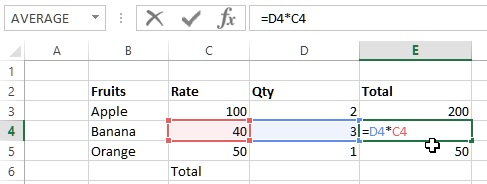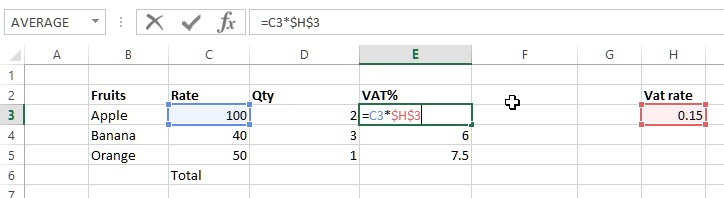# Cell references (Relative, Absolute, Mixed) in Excel

##### What are different types of cell references in MS Excel?

In previous post, we learnt about Functions and Formulas in Excel. In this post, we shall learn about different types of Cell references to be used in formulas.

### What is cell reference in Excel?

Cell reference is the name of a cell made by combining column and row names. It is used to refer a particular cell in the spreadsheet.

### Relative Cell Reference

The default cell reference in Excel is relative reference. Let's understand this by an example.

Write '=D3*C3' in E3 cell, this simply multiply the values of these cells and write. Now copy (either by dragging or copying) this formula to other cells (E4, E5).You would notice that even if we have just copied and pasted the formula to other cells, Excel basically takes the relative reference of it's left side cells and write value correct value into these cells.### Absolute Cell Reference

Generally, absolute cell reference in formula is given to that cell whose value is constant. Below example show VAT% in E column and that is the 0.15 of the Rate.

We have specified 'Vat Rate' in H3 cell and wrote '=C3*\$H\$3' to E3 cell. Absolute reference of row and column is given by prefixing '\$' symbol before row and column name.

Notice the '\$' symbol before column and row names.

This will calculate the 15% of 100 and write into E3 cell. If we copy this formula to E4 and E5 column then we get the correct value.and here is the result.QuickiesNow, assume that instead of giving Absolute reerence to H3 cell, you would have given Relative reference in the E3 cell. So your formula would have been '=C3*H3'. Now, if you copy-paste this to E4 and E5, you would have got '=C4*H4' and '=C5*H5' respectively and that will result in wrong value.

### Mixed Cell Reference

Mixed cell reference is the combination of Relateive and Absolute cell reference.

For example,

1. Formula '=C3*H\$3' says that we want Column H relative reference but Row 3 absolute reference.
2. Formula '=C3*\$H3' says that we want Column H absolute reference but Row 3 relative reference.
Views: 3094 | Post Order: 4